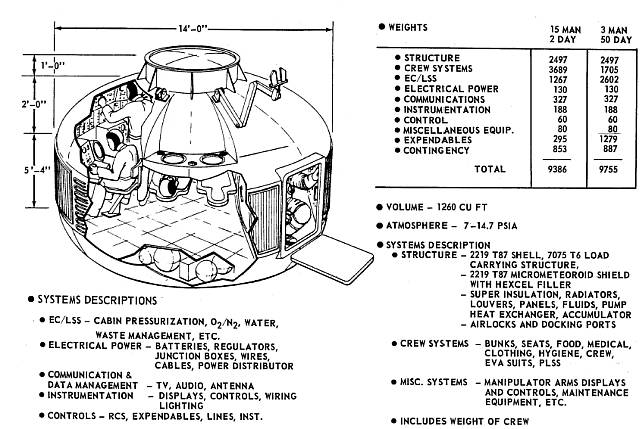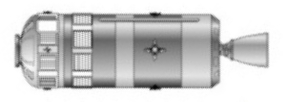## Pages

### THE ROCKET EQUATION

In this, the third of a four-part interconnected astronautics-based S.T.E.M. project, students will calculate the weight of the rocket propellant (both the fuel and the oxidizer) needed to conduct a space mission.

Students will also calculate the total weight of the Engine Module. The Crew Module is attached to the Engine Module to form a complete spacecraft.

Start Date
Third Quarter
Spring Semester

Time Frame

Mathematics Used
Natural Exponential Equations

Activating Previous Learning
Basic Mathematics
Scientific Calculator

Periapsis delta V (kps)
Apoapsis delta V (kps)
Delta V Budget (kps)
Transfer Time (days)
Mission Duration (days)

Crew Size (people)
Crew Module Weight (lbs)

::

Constants
Standard Gravity (km/s^2)
RL10 Rocket Engine Isp (sec)

Input
Rocket Inert Weight (lbs)
Propellant Mixture Ratio
Departure Orbital Altitude (km)
Destination Orbital Altitude (km)
Mission Duration (days)

Output
Rocket Exhaust Velocity (kps)
Rocket Empty Weight (kg)
Rocket Gross Weight (kg)
Total Amount of Propellant (kg)
Total Amount of LH2 (kg)
Total Amount of LO2 (kg)

::

Analysis
We will be using data from a spacecraft design that was completed but never constructed. The Boeing Space Tug study was finished in 1971. It called for a piloted rocket system that would operate in Low Earth Orbit (LEO). An un-piloted version of the rocket system would have carried satellites and other sensors to higher earth orbits.

This project will use the un-piloted section, or Engine Module (EM) of the system, which is displayed below.Engine Module (EM) DiagramEM Image

Combined with the Crew Module Image from Chapter 6,Command Module (CM) DiagramCM image

we now have a clear picture of what our spacecraft looks like:Spacecraft Image
This is the spacecraft that would have flown as designed in 1971. Our project will allow this great design to "fly" one more time.

::

We will be using the Rocket Equation to calculate the Propellant needed to go from one orbit to another.

The Rocket Exhaust Velocity (Vexh) is found by multiplying the Rocket Engine Specific Impulse (Isp) by the Standard Gravity.

The Inert Weight (M1) of the rocket includes the Empty Weight, the Reserve Propellant weight, and the Payload weight.

The Gross Weight (M0) of the rocket is the weight of the propellant plus M1.

We will use the delta V results found in Chapter 5.

::

The EM Diagram lists the Empty Weight as 5,610 lbs.

The diagram also lists the Propellant Weight at 39,800 lbs. We will assume a Propellant Reserve of 1% of the Total Propellant, or 398 lbs. Therefore our Usable Propellant is 39,402 lbs.

We need to make sure that we are carrying enough propellant to accomplish our mission. We can find this by substituting Propellant + M1 for M0.
delta V = Vexh * ln[(Propellant+M1)/M1] or
delta V = Vexh * ln(Propellant/M+ 1)
Dividing both sides by Vexh, we get,
delta V/Vexh = ln(Propellant/M+ 1)
Changing to exponential form (and switching sides) yields,
Propellant/M1 + 1 = e^(delta V/Vexh)
Subtracting one from both sides gives,
Propellant/M1 = e^(delta V/Vexh) - 1
Finally, multiplying both sides by Myields the Propellant Equation:

If the calculated Propellant is less than 39,402 lbs, then we can go.

Looking up the RL10 rocket specifications reveals a 5.85:1 Propellant Mixture Ratio; that is, for every part LH2, there is 5.85 parts of LO2. So for our spacecraft, we get,
LH2 = Total Propellant / (Propellant Mixture Ratio + 1)
LO2 = LH2 * Propellant Mixture Ratio
Therefore,
LH2 = 39,800 / (5.85 + 1) = 5,810 lbs
LO2 = 5,810 * 5.85 = 33,990 lbs

Note: The sample spreadsheet has the Project 4 information that is not needed for this project grayed-out. It is recommended that space is allowed for these future calculations.

Students that know how to use spreadsheet software should be encouraged to create their own app (remember, Google Apps are free!)

::

Teacher Lesson Plan
We will be using data from a spacecraft design that was completed but never constructed. The Boeing Space Tug study was finished in 1971. It called for a piloted rocket system that would operate in Low Earth Orbit (LEO). An un-piloted version of the rocket system would have carried satellites and other sensors to higher earth orbits.

INSERT TEACHER PRESENTATION HERE

::

The slides will guide the students as they run through the lesson powered by E^8:
1. Engage
• Lesson Objectives
• Lesson Goals
• Lesson Organization
2. Explore
• The Rocket Equation
• Engine Module Components and Definitions
3. Explain
• Basic Logarithmic Equations
• Engine Module Equation
• Engine Module Components and Equations
4. Elaborate
• Other Engine Modules
5. Exercise
• Calculating Engine Module Weight
• Engine Module Mission Scenario 1
• Engine Module Mission Scenario 2

6. Engineer
• The Engineering Design Process
• SMDC Spaceflight Plan
• Designing a Prototype
• SMDC Software
7. Express
• Displaying the SMDC
• Progress Report
8. Evaluate
• Post Engineering Assessment
This lesson can be delivered in one or two class periods, with students working on the project after school. It is recommended that a few minutes of a few class periods be set aside for student help.

The Student Workbook (below) accompanies the Teacher Lesson Plan.

INSERT TEACHER STUDENT WORKBOOK HERE
::

Missions
Spaceships are useless unless they have a place to go. These missions will add a sense of realism to the student project. Students will be placed into groups and asked to determine the total Crew Size and the total Spacecraft Weight for a given space mission:

INSERT TEACHER MISSIONS HERE

Other missions can be created and modified to suit the interest of the students. For example, a group that is interested in dinosaurs could be given a mission to find fossils on Mars. Or a group that wants to start their own business one day could get a mission to make a profit on placing a satellite in orbit.

Encourage students to design their own missions. This project is very flexible in that regard.

::

Presentation
Students will be asked to present their findings to the rest of the class. Parents are, of course, encouraged to attend (it is suggested that a pot luck would make things more interesting).

Each presentation will have slides that introduces the group, describes the mission, and displays the calculations. A short biography of the person named after the spacecraft should also be included.

Students that know how to use presentation software should be encouraged to create their own presentations (remember, Google Apps are free!)

::

Rubric
Students will also create a website and embed their slide presentation and their S.T.E.M. app in a webpage. Their journal will be kept on the webpage as well. If the class presentation is recorded to video, it can be uploaded to Youtube, then embedded in the webpage.

Therefore, each webpage (one for each project) should have the following items:
1. Embedded Slide Presentation
2. Embedded view of S.T.E.M. app
4. Link to working prototype of S.T.E.M. app
5. Journal Entries
6. Embedded Youtube video of the presentation
(Link opens in a new window)

This is not a scoring rubric; rather it is a guide of what is expected for the project.

The presentation should take between 5 and 10 minutes, unless there are a lot of questions from the audience. For a class with 6 groups, this comes out to between 30 and 60 minutes.

Students should be encouraged to dress professionally, and to practice their presentations beforehand.

Scoring and grading these projects is left up to the professionalism of the teacher.

::

Conclusion
The linear equations used in this project should be easy enough for the average high school Pre-Calculus student. The teacher may need to guide students through the setup of the equations and the calculations. As the semester progress, the concepts and the mathematics will become more challenging.

::

<<  |  TOP OF PAGE  |  >>